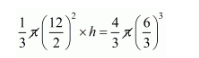# A solid metallic spherical ball of diameter 6 cm`
Question:

A solid metallic spherical ball of diameter 6 cm is melted and recast into a cone with diameter of the base as 12 cm. The height of the cone is

(a) 2 cm

(b) 3 cm

(c) 4 cm

(d) 6 cm

Solution:

Clearly,

The volume of recasted cone = volume of sphere$\frac{1}{3} \times 36 \times h=\frac{4}{3} \times 27$

$h=\frac{4 \times 27 \times 3}{3 \times 36}$

$h=3 \mathrm{~cm}$

Hence, the correct answer is choice (b).